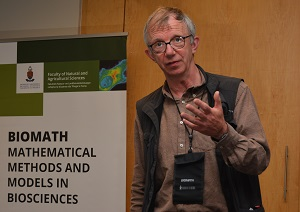# Estimating the mean of a small sample under the two parameter lognormal distribution

## Authors

• Peter Hingley European Patent Office

## Abstract

Lognormally distributed variables are found in biological, economic and other systems. Here the sampling distributions of maximum likelihood estimates (MLE) for parameters are developed when data are lognormally distributed and estimation is carried out either by the correct lognormal model or by the mis-specified normal distribution. This is designed as an aid to experimental design when drawing a small sample under an assumption that the population follows a normal distribution while in fact it follows a lognormal distribution.В Distributions are derived analytically as far as possible by using a technique for estimator densities and are confirmed by simulations. For an independently and identically distributed lognormal sample, when a normal distribution is used for estimation then the distribution of the MLE of the mean is different to that for the MLE of the lognormal mean. The distribution is not known but can be well enough approximated by another lognormal. An analytic method for the distribution of the mis-specified normal variance uses computational convolution for a sample of size 2. The expected value of the mis-specified normal variance is also found as a way to give information about the effect of the model misspecification on inferences for the mean. The results are demonstrated on an example for a population distribution that is abstracted from a survey.

## Author Biography

### Peter Hingley, European Patent Office